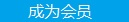您可以捐助，支持我们的公益事业。 1元 10元 50元 认证码：必填求知 文章 文库 Lib 视频 iProcess 课程 认证 咨询 工具 讲座 Modeler Code要资料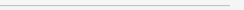订阅捐助
python 机器学习入门实战小结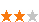378 次浏览     评价： 好 中 差
2019-8-1

 编辑推荐: 本文来自jianshu，本文使用机器学习入门经典例子泰坦尼克号乘客生存预测来实际操作，将对应的算法应用在给出的数据集上进行预测的详细过程。

Python 基础

3.1 系统环境

 import sys print("Python version: {}". format(sys.version)) import pandas as pd print("pandas version: {}". format(pd.__version__)) import matplotlib print("matplotlib version: {}". format(matplotlib.__version__)) import numpy as np print("NumPy version: {}". format(np.__version__)) import scipy as sp print("SciPy version: {}". format(sp.__version__)) import IPython from IPython import display print("IPython version: {}". format(IPython.__version__)) import sklearn print("scikit-learn version: {}". format(sklearn.__version__)) import random import time import warnings warnings.filterwarnings('ignore')

Python version: 2.7.10 (default, Oct 23 2015, 19:19:21)

[GCC 4.2.1 Compatible Apple LLVM 7.0.0 (clang-700.0.59.5)]

pandas version: 0.21.1

matplotlib version: 2.1.1

NumPy version: 1.13.3

SciPy version: 1.0.0

IPython version: 5.5.0

scikit-learn version: 0.19.1

3.11 加载机器学习库

 from sklearn import svm, tree, linear_model, neighbors, naive_bayes, ensemble, discriminant_analysis, gaussian_process from xgboost import XGBClassifier from sklearn.preprocessing import OneHotEncoder, LabelEncoder from sklearn import feature_selection from sklearn import model_selection from sklearn import metrics #可视化 import matplotlib as mpl import matplotlib.pyplot as plt import matplotlib.pylab as pylab import seaborn as sns from pandas.tools.plotting import scatter_matrix %matplotlib inline mpl.style.use('ggplot') sns.set_style('white') pylab.rcParams['figure.figsize'] = 12,8

3.2 数据观察

 data_raw = pd.read_csv('./Titanic/input/train.csv') data_val = pd.read_csv('./Titanic/input/test.csv') data1 = data_raw.copy(deep = True) data_cleaner = [data1, data_val] print (data_raw.info()) data_raw.sample(10) RangeIndex: 891 entries, 0 to 890 Data columns (total 12 columns): PassengerId 891 non-null int64 Survived 891 non-null int64 Pclass 891 non-null int64 Name 891 non-null object Sex 891 non-null object Age 714 non-null float64 SibSp 891 non-null int64 Parch 891 non-null int64 Ticket 891 non-null object Fare 891 non-null float64 Cabin 204 non-null object Embarked 889 non-null object dtypes: float64(2), int64(5), object(5) memory usage: 83.6+ KB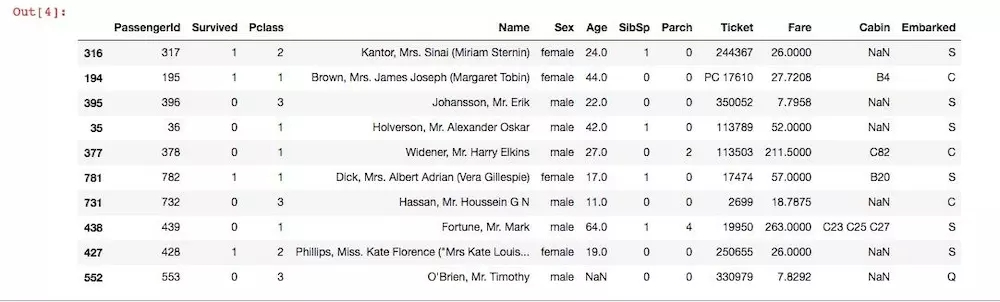3.3 数据修正、空值处理、新建特征、数据转换

** 修正清洗**：从上面的原始数据统计信息里可以看到，其中有很多错误或者不合理的数据，比如Age、Cabin那两列就有很多缺失值，还有如果数据中有一些奇异值，比如有一个乘客个年龄特别大，可能为300多甚至更大，这样的数据存在就可能会给我们的模型造成干扰，我们选择去修复这样的一些数据，是因为我们会认为数据修复之后对我们模型的精确性带来一定的提升，然而事实也缺失会是这样。

3.4 清理数据

 for dataset in data_cleaner: dataset['Age'].fillna(dataset['Age'].median(), inplace = True) dataset['Embarked'].fillna(dataset['Embarked'].mode(), inplace = True) dataset['Fare'].fillna(dataset['Fare'].median(), inplace = True) drop_column = ['PassengerId','Cabin', 'Ticket'] data1.drop(drop_column, axis=1, inplace = True) print(data1.isnull().sum()) print("-"*10) print(data_val.isnull().sum()) Survived 0 Pclass 0 Name 0 Sex 0 Age 0 SibSp 0 Parch 0 Fare 0 Embarked 0 dtype: int64 ---------- PassengerId 0 Pclass 0 Name 0 Sex 0 Age 0 SibSp 0 Parch 0 Ticket 0 Fare 0 Cabin 327 Embarked 0 dtype: int64

 #训练数据处理 for dataset in data_cleaner: dataset['FamilySize'] = dataset ['SibSp'] + dataset['Parch'] + 1 dataset['IsAlone'] = 1 dataset['IsAlone'].loc[dataset['FamilySize'] > 1] = 0 dataset['Title'] = dataset['Name'].str.split(", ", expand=True).str.split(".", expand=True) dataset['FareBin'] = pd.qcut(dataset['Fare'], 4) dataset['AgeBin'] = pd.cut(dataset['Age'].astype(int), 5) stat_min = 10 title_names = (data1['Title'].value_counts() < stat_min) data1['Title'] = data1['Title'].apply(lambda x: 'Misc' if title_names.loc[x] == True else x) print(data1['Title'].value_counts()) print("-"*10) #再看一下我们的数据 data1.info() data_val.info() data1.sample(10)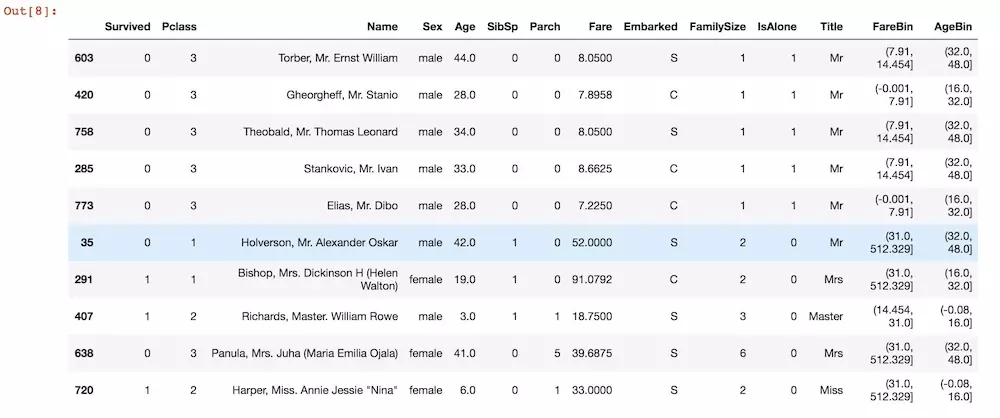3.5 格式转换

 label = LabelEncoder() for dataset in data_cleaner: dataset['Sex_Code'] = label.fit_transform(dataset['Sex']) dataset['Embarked_Code'] = label.fit_transform(dataset['Embarked']) dataset['Title_Code'] = label.fit_transform(dataset['Title']) dataset['AgeBin_Code'] = label.fit_transform(dataset['AgeBin']) dataset['FareBin_Code'] = label.fit_transform(dataset['FareBin']) Target = ['Survived'] data1_x = ['Sex','Pclass', 'Embarked', 'Title','SibSp', 'Parch', 'Age', 'Fare', 'FamilySize', 'IsAlone'] data1_x_calc = ['Sex_Code','Pclass', 'Embarked_Code', 'Title_Code','SibSp', 'Parch', 'Age', 'Fare'] data1_xy = Target + data1_x #print('Original X Y: ', data1_xy, '\n') data1_x_bin = ['Sex_Code','Pclass', 'Embarked_Code', 'Title_Code', 'FamilySize', 'AgeBin_Code', 'FareBin_Code'] data1_xy_bin = Target + data1_x_bin #print('Bin X Y: ', data1_xy_bin, '\n') data1_dummy = pd.get_dummies(data1[data1_x]) data1_x_dummy = data1_dummy.columns.tolist() data1_xy_dummy = Target + data1_x_dummy #print('Dummy X Y: ', data1_xy_dummy, '\n') data1_dummy.head()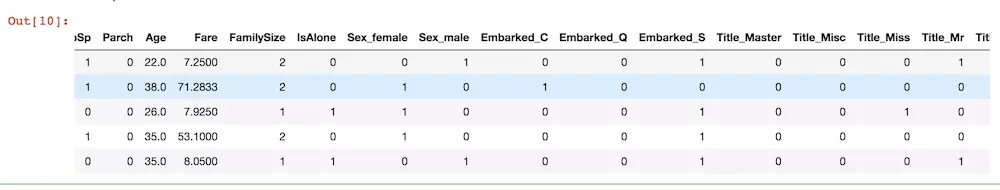3.6 拆分训练数据和测试数据

 #split the data set train1_x, test1_x, train1_y, test1_y = model_selection.train_test_split(data1[data1_x_calc], data1[Target], random_state = 0) train1_x_bin, test1_x_bin, train1_y_bin, test1_y_bin = model_selection.train_test_split(data1[data1_x_bin], data1[Target] , random_state = 0) train1_x_dummy, test1_x_dummy, train1_y_dummy, test1_y_dummy = model_selection.train_test_split (data1_dummy[data1_x_dummy], data1[Target], random_state = 0) print("Data1 Shape: {}".format(data1.shape)) print("Train1 Shape: {}".format(train1_x.shape)) print("Test1 Shape: {}".format(test1_x.shape)) train1_x_bin.head()

Data1 Shape: (891, 19)

Train1 Shape: (668, 8)

Test1 Shape: (223, 8)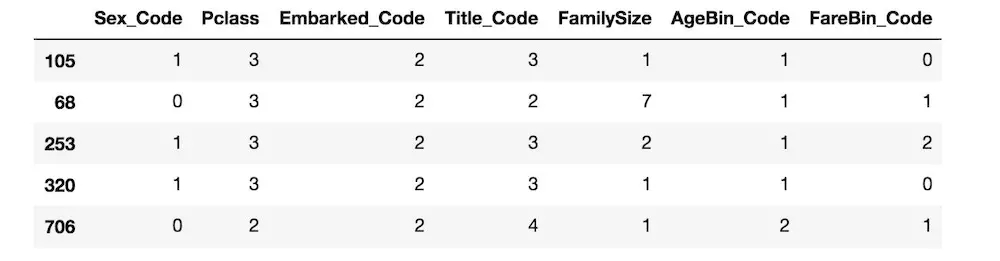3.7 可视化数据进行统计观测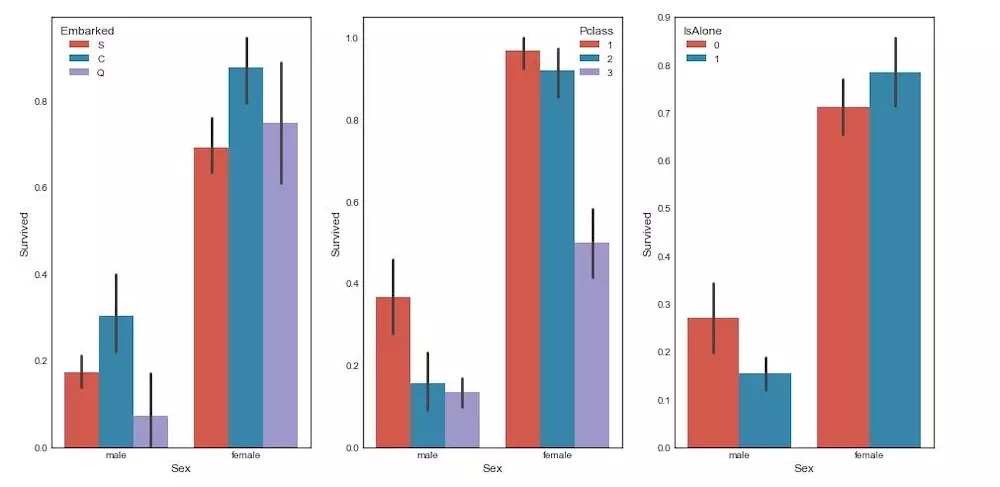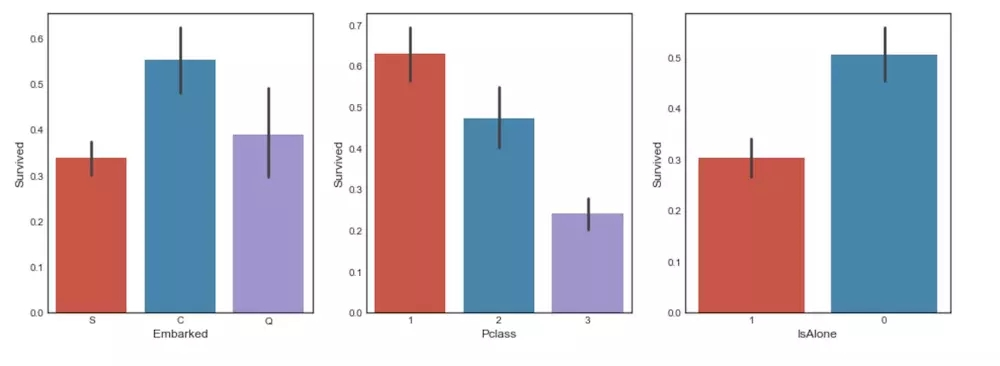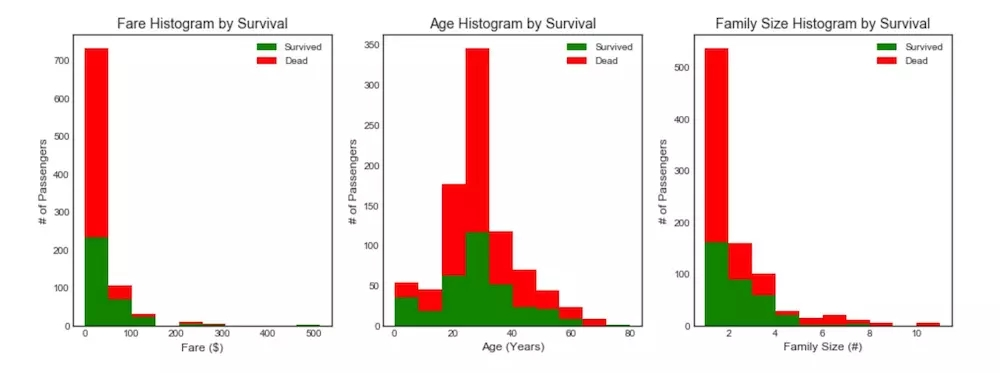data1整理后的数据协方差矩阵热力图3.7 建模分析

 MLA = [ ensemble.AdaBoostClassifier(), ensemble.BaggingClassifier(), ensemble.ExtraTreesClassifier(), ensemble.GradientBoostingClassifier(), ensemble.RandomForestClassifier(), gaussian_process.GaussianProcessClassifier(), linear_model.LogisticRegressionCV(), linear_model.PassiveAggressiveClassifier(), linear_model.RidgeClassifierCV(), linear_model.SGDClassifier(), linear_model.Perceptron(), #朴素贝叶斯 naive_bayes.BernoulliNB(), naive_bayes.GaussianNB(), neighbors.KNeighborsClassifier(), #支持向量机 svm.SVC(probability=True), svm.NuSVC(probability=True), svm.LinearSVC(), #决策树 tree.DecisionTreeClassifier(), tree.ExtraTreeClassifier(), discriminant_analysis.LinearDiscriminantAnalysis(), discriminant_analysis.QuadraticDiscriminantAnalysis(), XGBClassifier() ] #训练集拆分 cv_split = model_selection.ShuffleSplit(n_splits = 10, test_size = .3, train_size = .6, random_state = 0 ) #创建对比结果集 MLA_columns = ['MLA Name', 'MLA Parameters','MLA Train Accuracy Mean', 'MLA Test Accuracy Mean', 'MLA Test Accuracy 3*STD' ,'MLA Time'] MLA_compare = pd.DataFrame(columns = MLA_columns) MLA_predict = data1[Target] row_index = 0 for alg in MLA: #设置参数 MLA_name = alg.__class__.__name__ MLA_compare.loc[row_index, 'MLA Name'] = MLA_name MLA_compare.loc[row_index, 'MLA Parameters'] = str(alg.get_params()) #交叉验证 cv_results = model_selection.cross_validate(alg, data1[data1_x_bin], data1[Target], cv = cv_split) MLA_compare.loc[row_index, 'MLA Time'] = cv_results['fit_time'].mean() MLA_compare.loc[row_index, 'MLA Train Accuracy Mean'] = cv_results['train_score'].mean() MLA_compare.loc[row_index, 'MLA Test Accuracy Mean'] = cv_results['test_score'].mean() MLA_compare.loc[row_index, 'MLA Test Accuracy 3*STD'] = cv_results['test_score'].std()*3 alg.fit(data1[data1_x_bin], data1[Target]) MLA_predict[MLA_name] = alg.predict(data1[data1_x_bin]) row_index+=1 MLA_compare.sort_values(by = ['MLA Test Accuracy Mean'], ascending = False, inplace = True) MLA_compare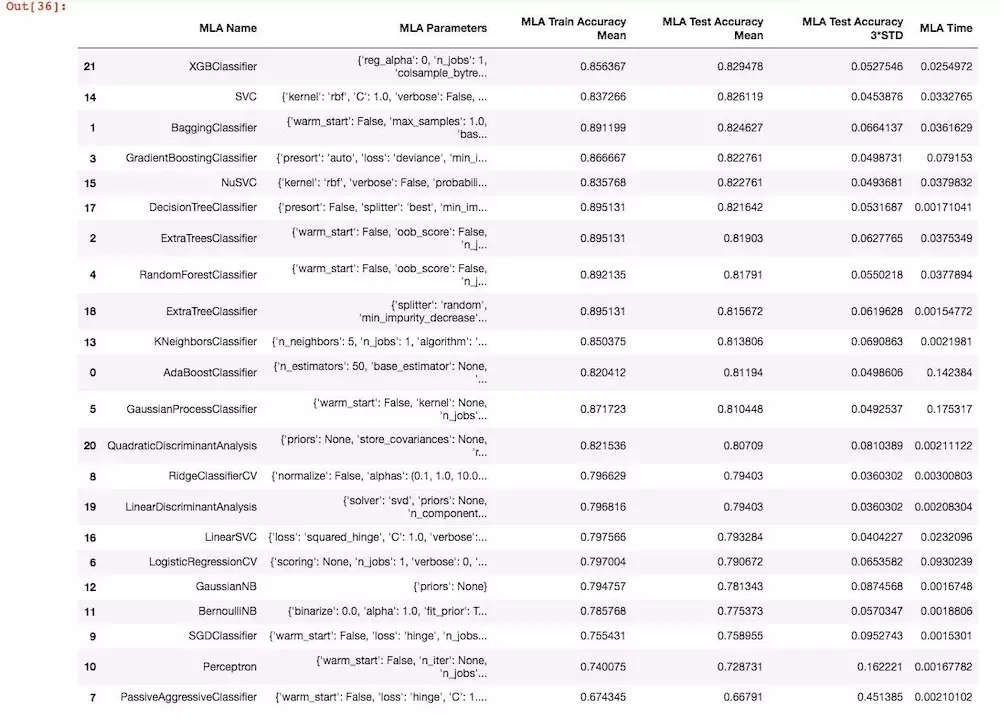3.8 小结分析378 次浏览  评价: 好 中 差订阅捐助
 相关文章 我们该如何设计数据库 数据库设计经验谈 数据库设计过程 数据库编程总结
 相关文档 数据库性能调优技巧 数据库性能调整 数据库性能优化讲座 数据库系统性能调优系列
 相关课程 高性能数据库设计与优化 高级数据库架构师 数据仓库和数据挖掘技术 Hadoop原理、部署与性能调优每天2个文档/视频 扫描微信二维码订阅
 订阅技术月刊 获得每月300个技术资源